﻿ 交通–电力暗挖隧道共建方案优化研究 Optimization on Co-Construction Scheme of the Traffic and Power Tunnel

Hans Journal of Civil Engineering
Vol. 08  No. 03 ( 2019 ), Article ID: 30315 , 10 pages
10.12677/HJCE.2019.83083

Optimization on Co-Construction Scheme of the Traffic and Power Tunnel

Ningqiang Shi1, Tingting Ge2, Xiaoguang Jing3*

1T.Y. Lin International Engineering Consulting (China) Co., Ltd., Chongqing

2Guangzhou College of South China University of Technology, Guangzhou Guangdong

3School of Civil Engineering, Chongqing University, Chongqing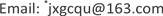Received: Apr. 29th, 2019; accepted: May 14th, 2019; published: May 21st, 2019ABSTRACT

Based on the theoretical analysis of the Jinzhuwan municipal traffic tunnel and power tunnel co-construction project of Tongmao Avenue in Chongqing, a two-dimensional finite element model is established for the proposed two construction schemes, and the traffic-electricity tunnel co-construction scheme is selected. The plastic zone radius of the rock, the stress at the elastoplastic interface and the elastoplastic displacement are compared and analyzed, and a better co-construction scheme is proposed.

Keywords:Co-Construction of Tunnels, Scheme Optimization, Theoretical Analysis, Numerical Simulation

1林同棪国际工程咨询(中国)有限公司，重庆

2华南理工大学广州学院，广东 广州

3重庆大学土木工程学院，重庆1. 引言

2. 共建方案的提出

3. 共建方案的理论分析

3.1. 等代圆法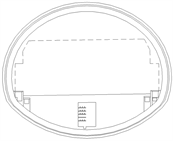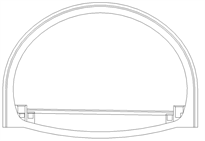(a) 方案一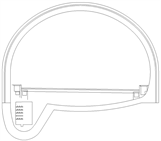(b) 方案二

Figure 1. The sketch of traffic-electric power co-constructed tunnel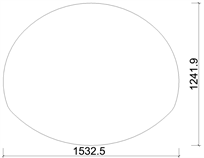(a) 方案一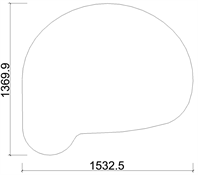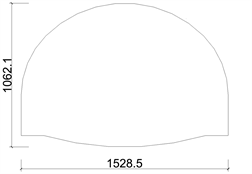(b) 方案二

Figure 2. Geometry traffic-electric power co-constructed tunnel

3.2. 优化方案的提出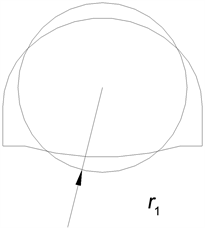(a) 右隧道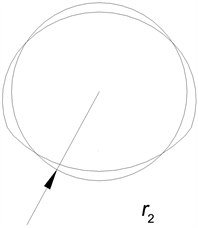(b) 方案一左隧道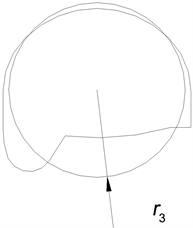(c) 方案二左隧道

Figure 3. Geometrical figure of equivalent circles

${R}_{0}={r}_{0}{\left[\left(1-\mathrm{sin}\phi \right)\frac{c\mathrm{cot}\phi +{\sigma }_{z}}{c\mathrm{cot}\phi +{p}_{\alpha }}\right]}^{\frac{1-\mathrm{sin}\phi }{2\mathrm{sin}\phi }}$ (1)

$\left\{\begin{array}{c}{\sigma }_{rp}=\left({p}_{\alpha }+c\mathrm{cot}\phi \right)\left(1-\mathrm{sin}\phi \right)\frac{c\mathrm{cot}\phi +{\sigma }_{z}}{c\mathrm{cot}\phi }-c\mathrm{cot}\phi \\ {\sigma }_{\theta p}=\left({p}_{\alpha }+c\mathrm{cot}\phi \right)\left(1+\mathrm{sin}\phi \right)\frac{c\mathrm{cot}\phi +{\sigma }_{z}}{c\mathrm{cot}\phi }-c\mathrm{cot}\phi \end{array}$ (2)

${u}_{{R}_{0}}^{e}=\frac{{R}_{0}^{2}\left(1+\mu \right)}{Er}\left({\sigma }_{z}-{\sigma }_{{R}_{0}}\right),r\ge {R}_{0}$ (3)

${u}^{p}=\frac{{R}_{0}^{2}\left(1+\mu \right)}{Er}\left({\sigma }_{z}-{\sigma }_{{R}_{0}}\right),{r}_{0}\le r\le {R}_{0}$ (4)

4. 共建方案优化的模拟分析

4.1. 模型的确定

4.2. 参数的选取Table 2. Physical mechanics parameter of the supporting structure

4.3. 施工工序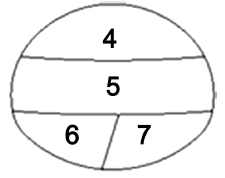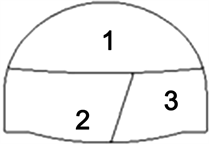(a) 方案一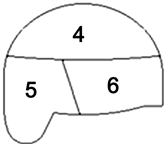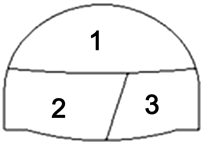(b) 方案二

Figure 4. Schematic diagram of the co-construction tunnel

4.4. 模拟结果及分析

1) 围岩应力特征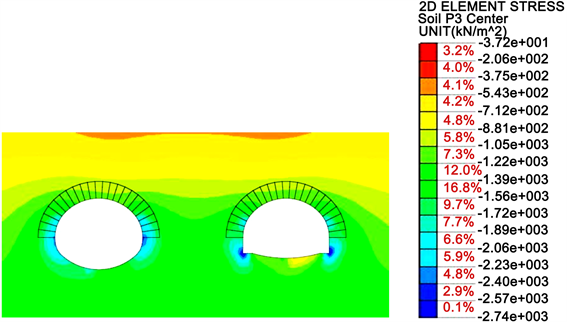(a) 方案一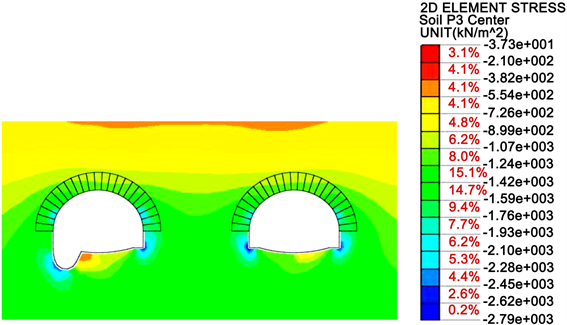(b) 方案二

Figure 5. Maximum principal stress diagram of tunnel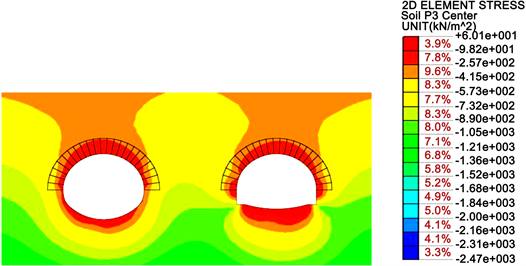(a) 方案一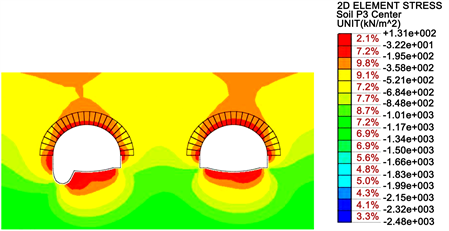(b) 方案二

Figure 6. Minimum principal stress diagram of tunnel

①方案一和方案二的最大主应力均为压应力，其最大值差别不大。方案一中应力集中主要发生在右隧道的拱脚和左隧道的拱腰处，其值分别达到为2.74 MPa和2.47 MPa；方案二中应力集中主要发生在左隧道和右隧道的拱脚处，其值均达到2.79 MPa。

②方案一和方案二的最小主应力中均出现了拉应力，两方案中的拉应力均分布在围岩的上部和隧道的拱底处。方案一中拉应力的最大值发生在右隧道的拱底处，其值约为0.06 MPa，方案二中其最大拉应力出现在电力隧道和仰拱连接处，约为0.13 MPa，此处易发生拉裂破坏。

③隧道的最大和最小主应力，总体上表现出拱脚最大，其次是拱腰，再次是拱顶和拱底。

2) 支护结构特征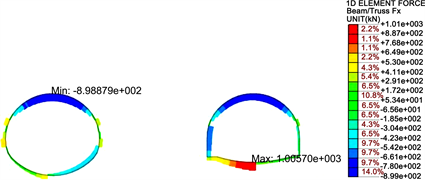(a) 方案一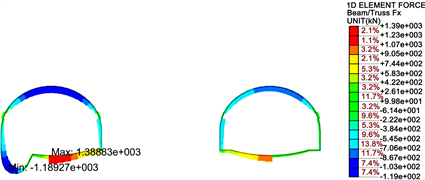(b) 方案二

Figure 7. Force of supporting structure of tunnel

3) 围岩位移特征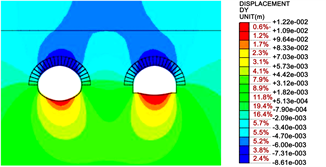(a) 方案一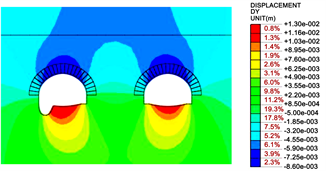(b) 方案二

Figure 8. Cloud map of vertical displacement diagram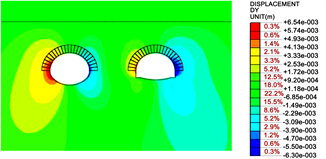(a) 方案一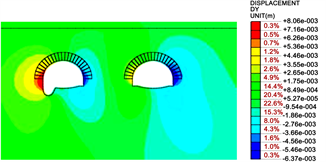(b) 方案二

Figure 9. Cloud map of horizontal displacement diagram

4) 围岩塑性区特征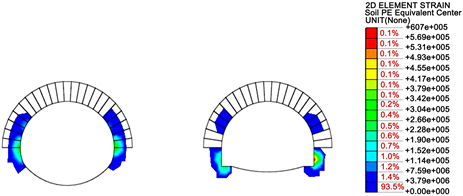(a) 方案一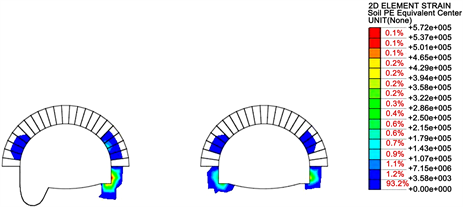(b) 方案二

Figure 10. Plastic zone of tunnel surrounding rock

5. 结论

Optimization on Co-Construction Scheme of the Traffic and Power Tunnel[J]. 土木工程, 2019, 08(03): 710-719. https://doi.org/10.12677/HJCE.2019.83083

1. 1. 张晓军. 隧道净距离上下交叠设计施工处治措施[J]. 公路交通技术, 2018, 34(5): 96-101.

2. 2. 王渭明, 王冲, 秦志斌, 等. 极小净距交叠隧道下行双线施工间距优化研究[J]. 现代隧道技术, 2017(5): 200-209.

3. 3. 台启民, 张顶立, 房倩, 等. 暗挖重叠地铁隧道地表变形特性分析[J]. 岩石力学与工程学报, 2014, 33(12): 2472-2480.

4. 4. 梅勇文. 明挖法施工城市隧道与综合管廊共建技术探讨[J]. 北方交通, 2018(6): 138-142.

5. 5. 阚绍德. 电力隧道工程与地铁工程共建设计浅析[J]. 广东土木与建筑, 2014(12): 53-55.

6. NOTES

*通讯作者。